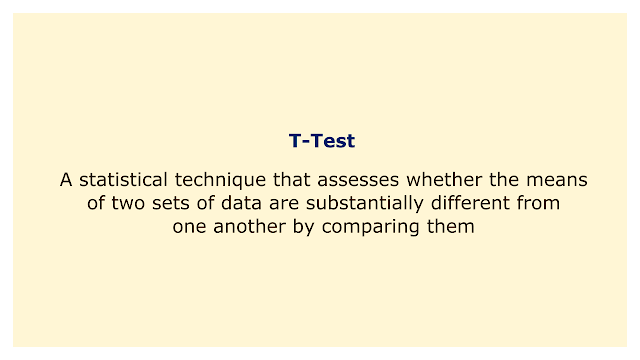# T-TestImage: Moneybestpal.com

### A t-test is a statistical technique that assesses whether the means of two sets of data are substantially different from one another by comparing them. It can be used to test a variety of financial hypotheses, such as whether a new investing strategy outperforms a benchmark, whether two stocks have different returns, or whether a portfolio has a positive alpha.

Depending on the type and number of groups being compared, there are various forms of t-tests. The most common types are:
• One-sample T-test: This type of Tt-test compares the mean of one set of data to a predetermined amount. A one-sample t-test, for instance, can be used to determine whether the average return on your portfolio is zero.
• Two-sample T-test: This t-test compares the means of two distinct sets of data. A two-sample t-test can be used, for instance, to determine whether the average returns of two different stocks are equal.
• Paired T-test: This t-test compares the means of two similar sets of data. A paired t-test can be used, for instance, to determine whether your portfolio's average returns before and after implementing a new strategy are equal.

A t-test also has two variants depending on whether you want to test for a specific direction or not:
• Two-tailed T-test: This variation determines whether there are any directional differences between the means of two groups. For instance, a two-tailed t-test can be used to determine whether stock A's average return differs from stock B's average return.
• One-tailed T-test: This variation determines whether the means of two groups differ in a certain direction, either more or less. To determine whether stock A's average return is higher than stock B's, for instance, you can use a one-tailed t-test.

To perform a t-test, you need three main pieces of information:
• The difference between the means of the two groups
• The standard deviation (or variance) of each group
• The sample size (or number) of each group

Depending on the kind and version of your t-test, you may use these numbers to compute a statistic called the t-value using a variety of formulas. The crucial value is then compared to this t-value, which is dependent on the degrees of freedom and level of significance you choose (often 5% or 0.05). (which depends on your sample size). The null hypothesis is rejected and you get to the conclusion that there is a substantial difference between the means if your t-value is higher than your critical value (in absolute terms). If not, you fail to rule out your null hypothesis and come to the conclusion that the means do not differ significantly.

A T-test is an easy and powerful tool for testing hypotheses in finance. However, it also has some limitations and assumptions that need to be considered before using it:
• It presumes that your data has a normal distribution (or approximately normal). If not, you might need to modify your data or use another non-parametric test, like the Wilcoxon rank-sum test.
• It presumes that each group in your data has an identical variance (or homogeneity). If not, you might need to modify your calculations or switch to another test, like Welch's T-test.
• It presumes that the data you provide is impartial both within and between groups. If not, you might have to take correlation into consideration or use a different design, like repeated measures ANOVA.
Tags